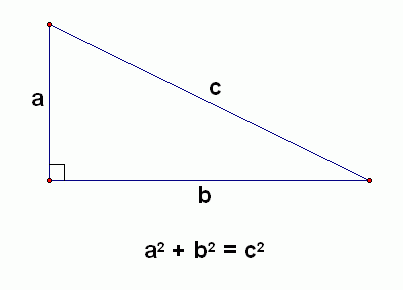# C++ 4. Creating a Pythagorean Theorem CalculatorIn part 3, you learned about variables and how to use your keyboard to assign the variables values. In this article we will create something useful with our new knowledge.

### Pythagorean Theorem Calculator

The Pythagorean Theorem states that the hypotenuse (c) is the sum of the squares of the other two sides (a and b). This can be written as , or, since we want to extract c we can write .Source: mathblog.com

The program below let's the user input a value for the two sides, then it calculates the hypotenuse based on the number it was fed, and finally outputs it to the screen for the user to read.

``````#include <iostream>
#include <string>
#include <math.h>
#include <cmath>

int main() {
float a;
float b;
float c;

std::cout << "Enter a value for a: ";
std::cin >> a;

std::cout << "Enter a value for b: ";
std::cin >> b;

float a2 = pow(a, 2);
float b2 = pow(b, 2);
c = sqrt(a2 + b2);

std::cout << "Hypotenuse: " << c << std::endl;
}``````

Output:

``Enter a value for a: 5Enter a value for b: 5Hypotenuse: 7.07107``

Pay attention the the first lines:

``````#include <iostream>
#include <string>
#include <math.h>
#include <cmath>``````

Remember in part 1 where I told you about these? We are including files in order to use specific functions. The library math.h is included here so we can use the function sqrt. The library cmath is included here so we can use the function pow.

``````	float a;
float b;
float c;``````

We have used the data type int to store integers, string to use sequences of characters, now we use float to handle floating points.

``````	std::cout << "Enter a value for a: ";
std::cin >> a;
std::cout << "Enter a value for b: ";
std::cin >> b;``````

We tell the computer to tell us to enter a value, then we tell the computer to let us enter a value, and then we enter a value. This happens two times, since we want to calculate the hypotenuse based on two sides.

``````	float a2 = pow(a, 2);
float b2 = pow(b, 2);``````

The function pow (for power) takes two parameters, base and exponent. In our case base: a and exponent: 2 for the first variable, and base: b and exponent: 2 for the second. The results are stored in new variables, a2 and b2.

``	c = sqrt(a2 + b2);``

This is now equal to the expression . The function sqrt (square root) takes one parameter. But wait a minute, we obviously put two numbers in the function above, what gives? Well, expressions are always calculated first in parameters. So we are really passing the sum  of a2 + b2 to the parameter, which is indeed only one number.

We can shorten the code above by reducing

``````	float a2 = pow(a, 2);
float b2 = pow(b, 2);
c = sqrt(a2 + b2);``````

to

``	c = sqrt(pow(a, 2) + pow(b, 2));``

They are equal.

``    std::cout << "Hypotenuse: " << c << std::endl;``

And here we are finally getting the result.

In the next article you will learn about memory concepts. Read C++ 5. Memory Concepts here.